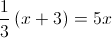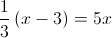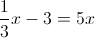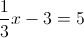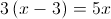## Example Questions

### Example Question #111 : How To Find The Solution To An Equation

If 5 + x is 5 more than 5,what is the value of 2x?

15

20

10

5

10

Explanation:

5 more than 5 = 10

5 + x = 10

Subtract 5 from each side of the equation: x = 5 → 2x = 10

### Example Question #112 : How To Find The Solution To An Equation

Let f(x) = 2x3 + 7x2 - 4x and g(x) = 4x - x3. If k is a positive number such that f(k) = 0, then what is g(k)?

2

1

7/8

17/8

15/8

15/8

Explanation:

First, we need to find the possible values of k such that f(k) = 0.

We can use the definition of f(x) to write an expression for k.

f(x) = 2x3 + 7x2 – 4x

f(k) = 2k3 + 7k2 – 4k = 0

In order to solve this equation, we will want to factor as much as we can. We can immediately see that we could take out a k from all three terms.

2k3 + 7k2 – 4k = 0

k(2k+ 7k – 4) = 0

Now, we need to factor 2k+ 7k – 4 by grouping. When we multiply the outer two coefficients we get (2)(–4) = –8. The middle coefficient is 7. This means we need to think of two numbers that multiply to give us –8, but add to give us 7. These two numbers are 8 and –1. We can now rewrite 2k+ 7k – 4 as follows:

2k+ 7k – 4 = 2k2 + 8k – k – 4

Group the first two terms and the second two terms.

2k2 + 8k – k – 4 = (2k2 + 8k ) + (–k – 4)

Next, factor out a 2k from the first two terms and a –1 from the last two terms.

(2k2 + 8k ) + (–k – 4) = 2k(+ 4) + –1(+ 4)

Then, factor out + 4 from the 2k and the –1.

2k(+ 4) + –1(+ 4) = (+ 4)(2k – 1)

Thus, 2k+ 7k – 4, when fully factored, equals (+ 4)(2k – 1).

Now, let's go back to the equation k(2k+ 7k – 4) = 0 and substitute (+ 4)(2k – 1) for 2k+ 7k – 4.

k(2k+ 7k – 4) = k(+ 4)(2k – 1) = 0

We now have three factors, and we can set each equal to zero to find the possible values of k.

The first factor is k. This means k = 0 is one value for k.

The next factor is k + 4.

k + 4 = 0

k = –4

The last factor is 2k – 1.

2k – 1 = 0

2k = 1

k = 1/2

The values of k for which f(k) = 0 are 0, –4, and 1/2. However, we are told that k is positive, so this means that k can only be 1/2.

Ultimately, the problem asks us to find g(k). This means we must use the equation for g(x) to find g(1/2).

g(x) = 4x – x3

g(1/2) = 4(1/2) – (1/2)3

= 2 – (1/8)

= 15/8

### Example Question #113 : How To Find The Solution To An Equation

In a group of 24 children, there are twice as many girls as there are boys.  How many boys are there?

12

14

10

16

8

8

Explanation:

Since there are twice as many girls as boys, we know that 2b = g.

Since there are 24 total, we know that b + g = 24.

Substituting the first equation into the second equation yields

b + 2b = 24

3b = 24

b = 8

### Example Question #114 : How To Find The Solution To An Equation

For each megawatt of power that it produces, a power plant spends $30,000 on coal,$50,000 on salaries, and $20,000 on general expenses. If the plant produces 250 megawatts, how much does it spend on salaries and general expenses combined? Possible Answers:$12,500,000

$15,000,000$17,500,000

$20,000,000 None of the other answers Correct answer:$17,500,000

Explanation:

The problem is simple if you do not mix up your values and their meanings. What we know is that for 250 megawatts, we should have 250 times the expenses in each of our categories. Therefore, for our data, we know the following.

For salaries, we have $50,000 * 250 or$12,500,000.

For general expenses, we have $20,000 * 250 or$5,000,000

Therefore, our total is: $12,500,000 +$5,000,000 = $17,500,000 We also could have calculated this by adding$50,000 and $20,000 together to get$70,000 per megawatt. This would be $70,000 * 250 =$17,500,000 as well.

### Example Question #115 : How To Find The Solution To An Equation

For what value of x does 4(3x – 2) = 12?

5/6

2/3

1

2

5/3

5/3

Explanation:

We have to solve the equation 4(3x – 2) = 12. First, we can distribute the left side.

4(3x) – 4(2) = 12

12x – 8 = 12

Then we add 8 to both sides.

12x = 20

Divide both sides by 12.

x = 20/12

Simplify 20/12 by dividing the numerator and denominator by 4.

x = 20/12 = 5/3

### Example Question #116 : How To Find The Solution To An Equation

If 11 + 3x is 29, what is 2x?

2

12

36

6

12

Explanation:

First, solve for x:

11 + 3= 29

29 – 11 = 3x

18 = 3x

x = 6

Then, solve for 2x:

2= 2 * 6 = 12

### Example Question #117 : How To Find The Solution To An Equation

If 2x = 3y = 6z = 48, what is the value of x * y * z?

3072

1024

1536

6144

2304

3072

Explanation:

Create 3 separate equations to solve for each variable separately.

1) 2x = 48

2) 3y = 48

3) 6z = 48

x = 24

y = 16

z = 8

* y * z = 3072

### Example Question #118 : How To Find The Solution To An Equation

If 3|x – 2| = 12 and |y + 4| = 8, then |x - y| can equal ALL of the following EXCEPT:

18

2

6

14

10

14

Explanation:

We must solve each absolute value equation separately for x and y. Remember that absolute values will always give two different values. In order to find these two values, we must set our equation to equal both a positive and negative value.

In order to solve for x in  3|x – 2| = 12,

we must first divide both sides of our equation by 3 to get |x – 2| = 4.

Now that we no longer have a coefficient in front of our absolute value, we must then form two separate equations, one equaling a positive value and the other equaling a negative value.

We will now get x – 2 = 4

and

x – 2 = –4.

When we solve for x, we get two values for x:

x = 6 and x = –2.

Do the same thing to solve for y in the equation |y + 4| = 8

and we get

y = 4 and y = –12.

This problem asks us to solve for all the possible solutions of |x - y|.

Because we have two values for x and two values for y, that means that we will have 4 possible, correct answers.

|6 – 4| = 2

|–2 – 4| = 6

|6 – (–12)| = 18

|–2 – (–12)| = 10

### Example Question #119 : How To Find The Solution To An Equation

If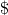is defined for all numbersandto be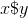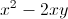, then what is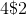?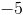Explanation:

In evaluating, we can simply plug in 4 and 2 forandrespectively. We then get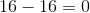.

### Example Question #120 : How To Find The Solution To An Equation

Translate into an algebraic expression:

One third of the difference of a number and 3 equals five times the number.